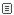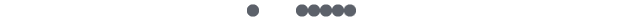##打通Python和C++之后,世界都和谐了

Python作为世界上最好的 胶水 语言(哼，世界上最好的语言当然是PHP==)，利用Python的简洁和C++的高效，基本可以解决99%的问题了吧~

1. 用C++扩展Python：当一个Python项目中出现了性能瓶颈时，将瓶颈部分抽离出来，用C++封装成一个Python可以调用的模块(so库);
2. 将Python内嵌入C++：当一个C++项目中有部分功能预期将会经常变更需求，期望获得更高的灵活性时，将这部分功能用Python实现，在C++中进行调用。这篇文章将简单介绍下第一部分的一种做法。Boost.Python

Boost作为一个大宝库，提供了我们所需要的这一功能。并且，在Boost的许多库中，已经默认使用了Boost.Python，所以也算是经过了充分的测试。

Boost的大部分功能都是以头文件的形式提供的，无需安装;但是也有少部分功能，需要进行手动编译。不幸，Boost.Python也是其中之一。

Hello World

++

`#include <boost/python.hpp>  char const* greet() {     return "hello, boost"; }  BOOST_PYTHON_MODULE(hello_boostpy) {     using namespace boost::python;     def("greet", greet); } `

`g++ -I /usr/include/python2.7/ -fPIC -shared -o hello_boostpy.so hello_boostpy.cc -lboost_python `

`# -*- coding: utf-8 -*- import sys sys.path.append('.')   def test():     import hello_boostpy     return hello_boostpy.greet()   if __name__ == "__main__":     print test() `

Expose Class

C++类：

++

`#include <boost/python.hpp> #include <vector> #include <string> #include <sstream> using namespace boost::python;  struct Person {     void set_name(std::string name) { this->name = name; }     std::string print_info();     void set_items(list& prices, list& discounts);               std::string name;     std::vector<double> item_prices;     std::vector<double> item_discounts; }; `

++

`void Person::set_items(list& prices, list& discounts) {     for(int i = 0; i < len(prices); ++i)     {         double price = extract<double>(prices[i]);         double discount = extract<double>(discounts[i]);         item_prices.push_back(price);         item_discounts.push_back(discount);     } } `

Python模块定义部分依旧是非常直观的代码：

`BOOST_PYTHON_MODULE(person) {     class_<Person>("Person")         .def("set_name", &Person::set_name)         .def("print_info", &Person::print_info)         .def("set_items", &Person::set_items)     ;    } `

`# -*- coding: utf-8 -*- import sys sys.path.append('.')   def test():     import person     p = person.Person()     p.set_name('Qie')     p.set_items([100, 123.456, 888.8], [0.3, 0.1, 0.5])     print p.print_info()   if __name__ == "__main__":     test() `

Py++评论发表评论